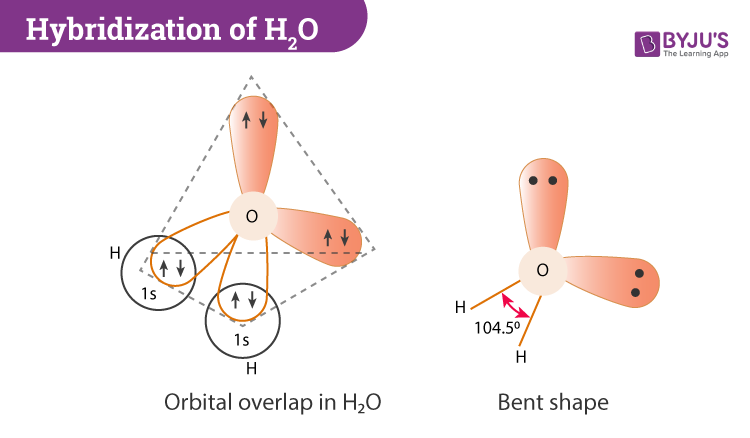# Hybridization of H2O (Water)

If we look at the general rule of hybridization it states that only the central atom undergoes the hybridization process. During the formation of a water molecule, we focus on the oxygen atom. In hybridization of H2O, the oxygen atom is sp3 hybridized.

 Name of the Molecule Water Molecular Formula H2O Hybridization Type sp3 Bond Angle 104.5o Geometry Angular or V-shaped

## What is the Hybridization of Water?

In this section, we will basically understand the formation of water on the basis of hybridization. The central atom here is oxygen which is hybridized. So if we observe the formation of the water molecule there are three 2p orbitals and one 2s orbital. These combine to create the four sp3 hybrid orbitals.Further, in the process, two-hybrid orbitals form covalent bonds with each hydrogen atom and two hybrid orbitals are occupied by lone pairs.

### Important Points to Remember

• In H2O hybridization orbitals having the same energy level will combine to form hybrid orbitals.
• The water molecule has two lone pairs and two bond pairs.
• Each O‒H covalent bond is called a sigma (σ) bond.

## H2O Molecular Geometry and Bond Angles

H2O has a tetrahedral arrangement of molecules or an angular geometry. This is mainly because the repulsion from the lone pair combination is more than bond-pair repulsion. Additionally, the existing pairs do not lie in the same plane. One pair is below the plane and the other one is above. This bond geometry is commonly known as a distorted tetrahedron.

As a result, the angle in a water molecule is 104.5° which again falls short of the true tetrahedral angle of 109°.